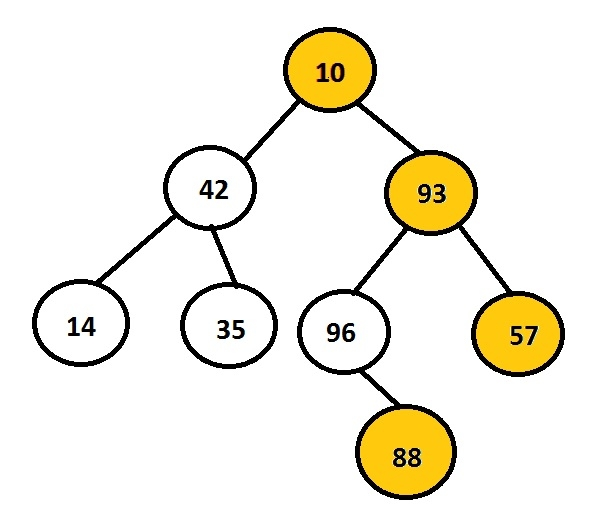# Print Right View of a Binary Tree in C language

The task is to print the right nodes of a given binary tree. Firstly user will insert data for creating a binary tree and than print right view of the tree so formed.The above diagram showcase the binary tree created with the nodes 10, 42, 93, 14, 35, 96, 57 and 88 amongst these nodes the one that lies in the right side of a tree are chosen and displayed over the screen. For example, 10, 93, 57 and 88 are the rightmost nodes of a binary tree.

## Example

Input : 10 42 93 14 35 96 57 88
Output : 10 93 57 88

There are two pointers associated with each node i.e. left and right. As per this question, program must traverse only right nodes. So, there is no need to bother about left child of a node.

Right view stores all those nodes that are the last nodes of their level. So, we can simply use recursive approach to store and access the nodes in such a way that right subtree is traversed before left subtree. Whenever the program detects the node whose level is greater than the previous node’s level than previous node is displayed as it will be the last node of its level.

The below code shows the c implementation of the algorithm given

## Algorithm

START
Step 1 -> create node variable of type structure
Declare int data
Declare pointer of type node using *left, *right
Step 2 -> create function for inserting node with parameter as item
Declare temp variable of node using malloc
Set temp->data = item
Set temp->left = temp->right = NULL
return temp
step 3 -> Declare Function void right_view(struct node *root, int level, int *end_level)
IF root = NULL
Return
IF *end_level < level
Print root->data
Set *end_level = level
Call right_view(root->right, level+1, end_level)
Call right_view(root->left, level+1, end_level)
Step 4 -> Declare Function void right(struct node *root)
Set int level = 0
Call right_view(root, 1, &level)
Step 5 -> In Main()
Pass the values for the tree nodes using struct node *root = New(10)
Call right(root)
STOP

## Example

#include<stdio.h>
#include<stdlib.h>
struct node {
int data;
struct node *left, *right;
};
struct node *New(int item) {
struct node *temp = (struct node *)malloc(sizeof(struct node));
temp->data = item;
temp->left = temp->right = NULL;
return temp;
}
void right_view(struct node *root, int level, int *end_level) {
if (root == NULL) return;
if (*end_level < level) {
printf("%d\t", root->data);
*end_level = level;
}
right_view(root->right, level+1, end_level);
right_view(root->left, level+1, end_level);
}
void right(struct node *root) {
int level = 0;
right_view(root, 1, &level);
}
int main() {
printf("right view of a binary tree is : ");
struct node *root = New(10);
root->left = New(42);
root->right = New(93);
root->left->left = New(14);
root->left->right = New(35);
root->right->left = New(96);
root->right->right = New(57);
root->right->left->right = New(88);
right(root);
return 0;
}

## Output

If we run above program then it will generate following output.

right view of a binary tree is : 10 93 57 88

Updated on: 22-Aug-2019

206 Views